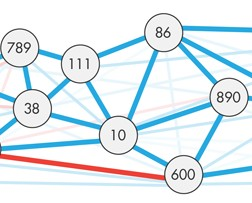# A surprising new proof is helping to connect the mathematics of infinity to the physical world

Natalie Wolchover in Quanta:With a surprising new proof, two young mathematicians have found a bridge across the finite-infinite divide, helping at the same time to map this strange boundary.

The boundary does not pass between some huge finite number and the next, infinitely large one. Rather, it separates two kinds of mathematical statements: “finitistic” ones, which can be proved without invoking the concept of infinity, and “infinitistic” ones, which rest on the assumption — not evident in nature — that infinite objects exist.

Mapping and understanding this division is “at the heart of mathematical logic,” said Theodore Slaman, a professor of mathematics at the University of California, Berkeley. This endeavor leads directly to questions of mathematical objectivity, the meaning of infinity and the relationship between mathematics and physical reality.

More concretely, the new proof settles a question that has eluded top experts for two decades…

More here.